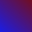Federated vs Non-Federated

The word "wiki" is a shortened form of "WikiWikiWeb." Since 1995 it has been used to describe a website on which many people collaborate, which we refer to here as a "Non-Federated Wiki" or "Original Wiki Model." Since about 2010, the term "wiki" has also been used to describe websites, each built by one person, which connect to one another in substantial ways, i.e. a "Federated Wiki." The descriptions and graphs below aim to illuminate the difference between these usages.

Original Wiki Model

In the original WikiWikiWeb and derivatives (e.g., Wikipedia), many people work on one thing (called a "wiki"). The collaboration happens inside, and in relation to, the "one thing".

digraph { layout=neato rankdir=BT overlap=false concentrate=false fontsize=9 bgcolor=lightblue // splines=" " "The Wiki (e.g.\nWikipedia)" [ color=red; pos = "0,0!"]; Serena -> "The Wiki (e.g.\nWikipedia)" Rafael -> "The Wiki (e.g.\nWikipedia)" Venus -> "The Wiki (e.g.\nWikipedia)" }

Federated Wiki Model

In Federated Wiki, each of many people works on their own website (called a "wiki"). The collaboration happens among the "many things," and they way people choose to link to one another's work.

digraph { layout=neato rankdir=BT overlap=false concentrate=false bgcolor=lightblue // splines=" " Serena [ pos="0,0"]; Rafael [ pos="5,0"]; Venus [ pos="2,2"]; "Serena's\nWiki" [pos="1,1"]; "Rafael's\nWiki" [pos="4,1"]; "Venus's\nWiki" [pos="3,3"]; "Serena's\nWiki" [color=red] "Venus's\nWiki" [color=red] "Rafael's\nWiki" [color=red] Serena -> "Serena's\nWiki" Venus -> "Venus's\nWiki" Rafael -> "Rafael's\nWiki" "Rafael's\nWiki" -> "Venus's\nWiki" -> "Serena's\nWiki" -> "Rafael's\nWiki" "Venus's\nWiki" -> "Rafael's\nWiki" -> "Serena's\nWiki" -> "Venus's\nWiki" }

A way they can connect

This graph sketches out an idea of how content could be generated in Federated Wiki, and then migrated to a central wiki like Wikipedia.

digraph { layout=circo rankdir=BT overlap=false concentrate=false bgcolor=lightgreen label="\n\n Relating FedWikis to Wikis" // splines=" " //Serena [ pos="0,0"]; //Rafael [ pos="5,0"]; //Venus [ pos="2,2"]; //"Serena's\nWiki" [pos="1,1"]; //"Rafael's\nWiki" [pos="4,1"]; //"Venus's\nWiki" [pos="3,3"]; "Serena's\nWiki" [color=blue] "Venus's\nWiki" [color=yellow] "Rafael's\nWiki" [color=green] Serena -> "Serena's\nWiki" Venus -> "Venus's\nWiki" [style=dotted] Rafael -> "Rafael's\nWiki" "Rafael's\nWiki" -> "Venus's\nWiki" -> "Serena's\nWiki" -> "Rafael's\nWiki" "Venus's\nWiki" -> "Rafael's\nWiki" -> "Serena's\nWiki" -> "Venus's\nWiki" {"Serena's\nWiki" "Rafael's\nWiki" "Venus's\nWiki"} -> "The Wiki (e.g.\nWikipedia)" [style=dotted penwidth=3] "The Wiki (e.g.\nWikipedia)" [ color=red; penwidth=3] }

Pete Kaminski's version of basically the same diagram: https://chat.collectivesensecommons.org/files/1zqxennsx7n93xmi5j7eitdm3w/public?h=KTq5zSJ-uGDho3k6J35uhpJA0SS0iIW_fa17-ew2Sv8